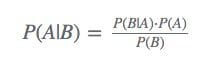Topics: AI | Data Science | Data Visualization | Deep Learning | Machine Learning | NLP | Python | R | Statistics

KDnuggets Home » News » 2016 » Feb » Tutorials, Overviews » Bayes Theorem for Computer Scientists, Explained ( 16:n06 )

# Bayes Theorem for Computer Scientists, Explained

Data science is vain without the solid understanding of probability and statistics. Learn the basic concepts of probability, including law of total probability, relevant theorem and Bayes’ theorem, along with their computer science applications.

### Bayes’ theorem

Bayes’ theorem is much more powerful — it allows us to understand things about the known world based on the unknown world when we have enough information relating the two. Let’s pick up where we left off in the last problem. We still know everything that was given and now we know some more information based on the law of total probability.

Let’s consider now that we chose a random person from this room, and that the chosen person’s favorite color is green (event B). In this example, however, we don’t yet know the sex of person. With Bayes’ theorem, we can answer the question “Given that a randomly selected person likes green, what is the probability that the person is a female?” Below is an updated figure based on our new knowledge of the situation.In other words, we know the person was picked within the green circle. What is the probability that the person was picked from the red shaded area? How would you compute this without any notion of probability theory?

My approach would to be to take the ratio of the red area with respect to the entire green area — and that is the approach that Bayes’ theorem takes as well. From the previous work, we know that the amount of women in the green circle (given 100 people) is 70⋅0.4=28 people. Furthermore, we know that the amount of people in the circle is 50.5 people. So the probability that a female was picked from the people that liked green is 28/50.5≈0.55 or 55%.

Formally, Bayes’ theorem is expressed asHowever, the key to really understanding Bayes’ theorem is recognizing that the denominator is actually just the law of total probability! So the equation can also be expressed this wayThink of Bayes’ formula this way: the numerator is the section in the green circle that we are focused on, and the denominator is all of the pieces of the green circle (including the piece we are looking at) summed together. So Bayes’ theorem is just a ratio.

Bonus

the way I think of Bayes’ theorem is

P(A|B)=focused piece of circle focused piece of circle+rest of the circle pieces### Application to Computer Science

Briefly, Bayes’ theorem is the foundational theory in the field of Bayesian inference. After establishing a firm method between relating the outcome of a know event in terms of an unknown event, we can now observe the relationship between the two events (and vice-versa). Using Bayes’ rule, we can update our knowledge about how these two events are related. These ideas belong to a broader school of thought called Bayesian statistics which helps us build advanced statistical models using techniques like Markov Chain Monte Carlo methods and the No-U-Turn sampler. If you would like to try these techniques out, I recommend you use an open source library like PyMC3 instead of coding one up yourself.

### Conclusion

There are many other foundational concepts not covered here like the Union-bound theorem (Boole’s inequality) and the Inclusion-exclusion principle. However, these concepts are mostly useful for building the theorems (including the ones laid out in this article) and not in many practical applications. With a strong understanding of Bayes’ theorem, you are in a good position to dive into the deeper field of probabilistic programming.

Bio: Clay McLeod is writing masters’ thesis on the subject of malleability in deep neural networks — the benefits and detriments of giving a deep neural network more trainable parameters.

Related:

Top Stories Past 30 Days
Most Popular
Most SharedGet KDnuggets, a leading newsletter on AI, Data Science, and Machine Learning